## Recommended Posts

Dear community!

I just stumbled upon a strange behavior of the Gibbs free enthalpy when fiddling about with Gibbs Helmholtz equation which I cannot explain. The first formula in the attached file is the one that I've been using all the time, the derivative of G with respect to temperature at constant pressure. The second one just came to my mind when using an alternative derivative. As cp of the reaction is, generally speaking, not equal to zero for reactions with no change in pressure, it seems that these two formulas contradict each other. Surely that cannot be. Anyone got a solution that resolves that contradiction? Thanks in advance.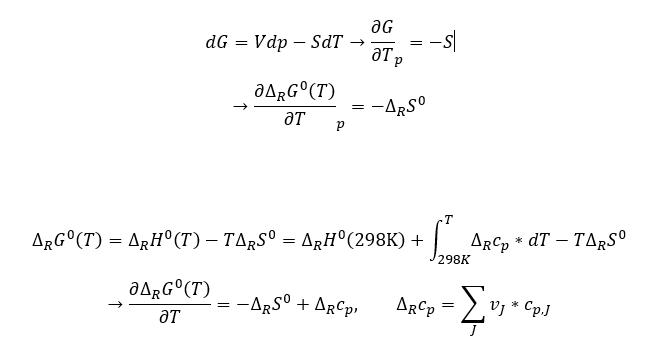##### Share on other sites
Posted (edited)
47 minutes ago, Matthew99 said:

Dear community!

I just stumbled upon a strange behavior of the Gibbs free enthalpy when fiddling about with Gibbs Helmholtz equation which I cannot explain. The first formula in the attached file is the one that I've been using all the time, the derivative of G with respect to temperature at constant pressure. The second one just came to my mind when using an alternative derivative. As cp of the reaction is, generally speaking, not equal to zero for reactions with no change in pressure, it seems that these two formulas contradict each other. Surely that cannot be. Anyone got a solution that resolves that contradiction? Thanks in advance.

<image>

Maybe I have to look at it closer, but seems to me there's something wrong there.

There's no such thing as Gibbs free enthalpy, but Gibbs free energy.

Edit: Sorry, I changed F with G. I erased my equations. I'll think about it a bit longer.

What's the sub R?

Incrementing G:

$G=U+PV-TS=H-TS$

should give you,

$\triangle G=\triangle H-T\triangle S-S\triangle T$

and not,

$\triangle G=\triangle H-T\triangle S$

Could that be the problem?

I don't know what the deltas represent (processes?, balance from products to reactants?) In any case I think that could be the problem.

Edit 2: On second thought, you seem to be considering fixed temperature, right?

Edit 3: You're taking increments at constant (standard conditions) temperature, yet you're integrating in T.

I'm confused. Is temperature changing or not?

Edited by joigus

##### Share on other sites
Posted (edited)

First of all, I meant gibbs free energy, not gibbs free enthalpy, that was a mistake that occurred due to translation as I am learning physical chemistry in german and it is called free enthalpy there.

Secondly, sub R stands for reaction - for example, G sub R would mean the molar free energy of products minus reactants. When the formula is valid for one side of the equation it must be for the whole equation too.

3rd, the total differential of G should indeed be the formula that I used in the beginning, I'll post the calculation via legendre transform of H down below.

Concerning Edit 2/3: The superscript zero should suggest that the reaction happens at standard conditions in terms of standard pressure and equal concentrations of reactants/products. H sub r therefore is the enthalpy of the reaction as a function of temperature (as reactants and products can have different heat capacities, the enthalpy of the reaction can vary with different temperatures).

Edit: The formula should be valid for fixed temperatures, however, as the temperature chosen can be anything and the total enthalpy is defined by standard conditions at 298K, we have to integrate from 298 to T

Edited by Matthew99

##### Share on other sites
Posted (edited)

Hi, Matthew. Sorry for the misunderstanding. Now I understand much better. The last identities for dH in terms of (V, P, T, S) or dG in terms of (V, P, S, T) are totally right AFAICT. The problem I see is in delta G in terms of (H, T, S) in an intermediate step that I think is the one that leads to contradiction.

You write,

$\triangle_{R}G^{0}\left(T\right)=\triangle_{R}H^{0}\left(T\right)-T\triangle_{R}S^{0}$

(this is the one that's wrong, I think)

Then you take the delta H^0 at T to delta H^0 at 298K by,
$\triangle_{R}H^{0}\left(T\right)=\triangle_{R}H^{0}\left(298K\right)+\int_{298K}^{T}dT\left(\triangle_{R}c_{p}\right)$
The last step is right, but the mistake, I think, is in the first equation, as,
$\triangle_{R}G^{0}\left(T\right)\neq\triangle_{R}H^{0}\left(T\right)-T\triangle_{R}S^{0}$
When the actual identity should be,
$\triangle_{R}G^{0}\left(T\right)=\triangle_{R}H^{0}\left(T\right)-T\triangle_{R}S^{0}-S^{0}\triangle_{R}T$
The term you're missing in this intermediate step is the one that cancels the discrepancy, if I'm not mistaken.
Edit: Thanks for the careful explanation. +1. I hope that helps.
Edited by joigus

##### Share on other sites

Thanks a lot for the detailed answer, however, from my point of view, this does not cancel the discrepancy. I forgot to mention that S*deltaT is usually ignored here (at least in Atkins physical chemistry, they say that as the change in system temperature is very low in open systems, we can ignore the last term). On the other hand, the integration over the heat capacity of the reaction from 298K to T takes into account that the reaction enthalpy at temperature T can be different that the standard reaction ethalpy, which is defined at 298K.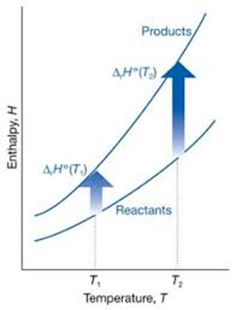##### Share on other sites
10 minutes ago, Matthew99 said:

Thanks a lot for the detailed answer, however, from my point of view, this does not cancel the discrepancy. I forgot to mention that S*deltaT is usually ignored here (at least in Atkins physical chemistry, they say that as the change in system temperature is very low in open systems, we can ignore the last term). On the other hand, the integration over the heat capacity of the reaction from 298K to T takes into account that the reaction enthalpy at temperature T can be different that the standard reaction ethalpy, which is defined at 298K.

Is this the beginning of the section in Atkins you are looking at?

##### Share on other sites

Hello studiot,

I've got another version so there are some differences but the involved chapter is called chemical equilibrium. The formula I posted (the first one) comes into action when they derive the gibbs-helmholz equation.

##### Share on other sites
16 minutes ago, Matthew99 said:

as the change in system temperature is very low in open systems, we can ignore the last term

Well, that's good to know. Thank you. Now I think some questions are in order.

Very low with respect to what? There are no absolute standards to say that something is low, AFAIK.

Low it may be, but apparently, not enough as to consider,

$\int_{298K}^{T}dT\left(\triangle_{R}c_{p}\right)$

as negligible. Why can you consider the temperature fixed as to some effects and varying as to other effects? Could you be more specific, please?

I really want to help, if it's possible. Maybe it's not.

##### Share on other sites

Well, I suppose this is valid for reactions that happen under normal conditions and in large systems, I haven't seen any calculation where S*deltaT isn't neglected yet. The deltaT here stands for the temperature change that the system undergoes when the reaction happens, and this is usually low compared to the absolute temperature of the system. On the other hand, the integral you just posted is something completely different. This is the difference in reaction enthalpy with respect to standard reaction enthalpy at 298. When the reaction happens at temperature T, the enthalpy of the reaction can be higher/lower depending on the heat capacity of reactants/products than it would be when it would happen at 298K (where H0 is defined). When you take a close look at the picture I posted in my 3rd post, this is the graphical representation of what I'm trying to explain.

##### Share on other sites
Posted (edited)
45 minutes ago, Matthew99 said:

Hello studiot,

I've got another version so there are some differences but the involved chapter is called chemical equilibrium. The formula I posted (the first one) comes into action when they derive the gibbs-helmholz equation.

The G-H eqaution is derived for conditions of constant temperature and an infinitesimal change of temperature.
I don't see anything like yours in either my 5th or 6th Atkins.

The relationship to enthalpy comes in as the work term w'

Gibbs-Helmholtz (original equation)

$\Delta H + w' = T{\left( {\frac{{\partial w'}}{{\partial T}}} \right)_P}$

but

$w' = - \Delta G$

So

$\Delta H - \Delta G = - T{\left( {\frac{{\Delta G}}{{\partial T}}} \right)_P}$

Is the form of the equation in Atkins.

Edited by studiot

##### Share on other sites
Posted (edited)
2 hours ago, Matthew99 said:

When you take a close look at the picture I posted in my 3rd post, this is the graphical representation of what I'm trying to explain.

Sorry, I wasn't able to look at the picture with any degree of closeness without my eyes hurting --it's quite blurry. Now I can intuit what it is about.

I think I'm on to it. It took me a while to realize what you meant. I think the problem is quite subtle. There must be a mathematical reason why you can't exchange the "operators"

$\triangle_{R}$

which compares two phases of the thermodynamic system that are coexisting

and,

$\left(\frac{\partial}{\partial T}\right)_{P}$

which obtains the infinitesimal change by T in an infinitesimally small reversible change (we physicists use the word "irreversible" in a different sense). The most "suspicious" expression is the second one, in which you use an integral. Unless someone comes up with the answer I'd love to keep thinking about it tomorrow, when I find the time.

Does that make sense?

PD: Of course, when you write:

$\triangle_{R}c_{p}=\sum_{J}\nu_{J}c_{p,J}$

you mean,

$\sum_{\textrm{products}}\nu_{j}c_{p,j}-\sum_{\textrm{reactants}}\nu_{i}c_{p,i}$

right?

Just one caveat: You're trying to solve a logical contradiction between 2 expressions, one of which involves an approximation. It could take some time.Edited by joigus

##### Share on other sites

@joigus Sorry, I forgot to mention that v_J is negative for reactants and positive for products, that's some notation the Atkins has.

@studiot I do not know where it is in the 5/6th edition, but in the 9th edition, there is a chapter called standard molar gibbs energies where the formula I used is introduced: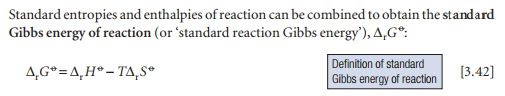Obviously, as joigus pointed out, this is only exactly the case when change in system temperature due to the reaction is constant, however, I've seen that this equation is commonly used for reactions in open systems as the change of system temperature per mole reacting is quite small compared to absolute system temperature.

Lets take a closer look at this formula.

As far as I understood, the superscript zero at G only indicates that products and reactants appear in same proportions, as the reaction Gibbs energy changes with the reaction quotient because the entropy of reaction is a function of the reaction quotient: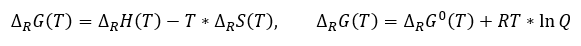When Q is 1, the reaction Gibbs energy is equal to the standard reaction gibbs energy, regardless of temperature. This is also stated in Atkins: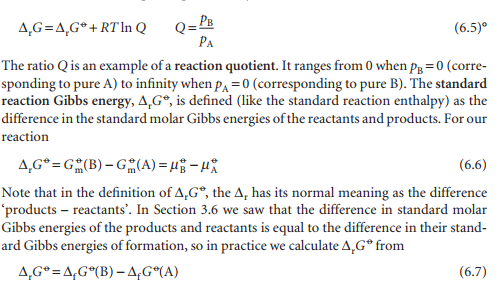As far as I know, the equation on the left does not make sense to use because finding the reaction entropy for any given reaction quotient seems difficulty. Thus, usually equation 3.42 is used to calculate the standard reaction Gibbs energy because we know the standard reaction entropy. Furthermore, the enthalpy of reaction should not change with reaction quotient (at least as far as I know). Therefore, this should be valid: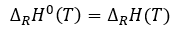So, lets consider a simple reaction with reactant A and product B: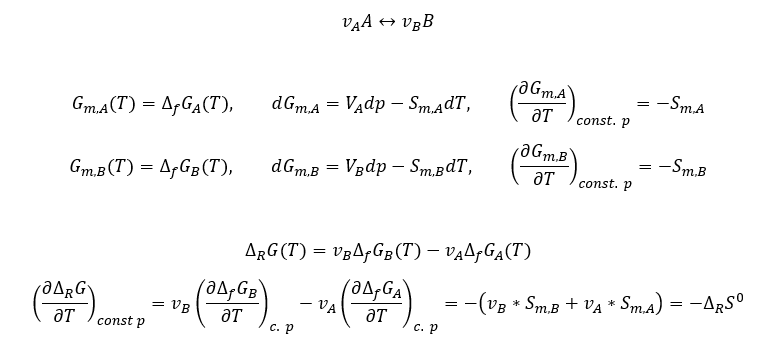Now, a different approach: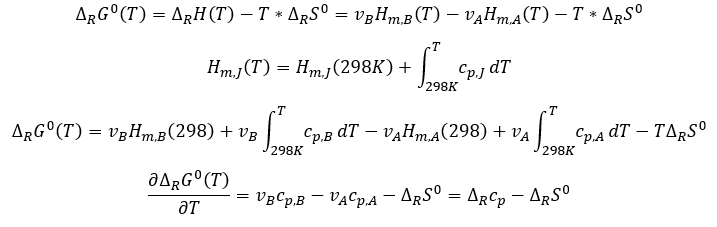This is the equation I posted in the first place and the results I get from these two approaches do not mach up. I can't see any mathematical / logical error in my calculations.

##### Share on other sites
Posted (edited)
8 hours ago, Matthew99 said:

This is the equation I posted in the first place and the results I get from these two approaches do not mach up. I can't see any mathematical / logical error in my calculations.

Thank you for posting more detail.

8 hours ago, Matthew99 said:

I do not know where it is in the 5/6th edition, but in the 9th edition, there is a chapter called standard molar gibbs energies where the formula I used is introduced:

The material is still there, much of it in the same words in all these editions, except that in my 5th ed (which I think is the best one) there is a warning additional to the excerpt you posted.

"Note that in the definition of ΔrG0 ,  the Δr has the normal meaning as the difference products - reactants."

The two pages I posted hold what I think is the key to your dilemma.
If you look at the middle of the second page you will see the warning

"We must be careful to distinguish the reaction Gibbs energy, the slope of G at a specified composition, from the standard molar reaction Gibbs energy  ΔrG0

In this case Δr  has its normal meaning of the difference of two quantities."

Reading both the two pages I posted explain that one of two similar sounding quantities is a a slope of a graph (of G v an extent of reaction parameter), the other is a difference in values of G itself.

As a matter of interest I cannot get Latex to offer the london underground (strikethrough zero) symbol but +1 to Scienceforums as I can manufacture it with the superscript and strikethrough tools on the site editor.

Edited by studiot

##### Share on other sites
Posted (edited)

Dear studiot!

I see what you wanted to point out, however, I do not think that this resolves the problem. As it says, standard reaction gibbs energy is the slope at xi = 0. Nevertheless, the slope is still a function of temperature:Therefore,I am not changing xi , I only want to quantify how much the reaction gibbs energy depends on T.

Edited by Matthew99

##### Share on other sites
Posted (edited)
19 hours ago, Matthew99 said:

I am not changing xi , I only want to quantify how much the reaction gibbs energy depends on T.

On 6/18/2020 at 8:21 AM, Matthew99 said:

Furthermore, the enthalpy of reaction should not change with reaction quotient (at least as far as I know). Therefore, this should be valid:

I think that buried in all that maths are some conflicting assumptions so let me offer you an alternative.

The Gibbs-Helmhotz equation is not one equation  -  it is a bunch of equations and part of the trick is to pick the right one for your purposes.

Usually this involves using a form that converts a quantity that you know or can easily determine by measurement into a quantity that you don't know but want to.

You also need to use a form that offers an alternative extensive (intensive) variable to substitute for your unknown extensive (intensive) variable.

You need both extensive and intensive variables to multiply together to get the units of work/energy.

So here is one form of GH connecting the free energy of a reaction to the heat of reaction and temperature.

$\Delta H = \Delta G - T{\left( {\frac{{\partial \Delta G}}{{\partial T}}} \right)_P}...........1$

This is easy to derive, are you happy with that?

In state variable form this can also be written without the difference operator delta which gives the ex tensive quantity as a difference (products - reactants).

$H = G - T{\left( {\frac{{\partial G}}{{\partial T}}} \right)_P}..................2$

here are a few more that I will not use for this question, but may be useful elsewhere.

$U = A - T{\left( {\frac{{\partial A}}{{\partial T}}} \right)_V}$

$U = H - P{\left( {\frac{{\partial H}}{{\partial T}}} \right)_S}$

$H = G - T{\left( {\frac{{\partial G}}{{\partial T}}} \right)_p}$

$A = G - P{\left( {\frac{{\partial G}}{{\partial P}}} \right)_T}$

$G = A - V{\left( {\frac{{\partial A}}{{\partial P}}} \right)_T}$

Returning to equation 1 dividing through by T2 and rearranging we have

$\frac{1}{T}{\left( {\frac{{\partial \Delta G}}{{\partial T}}} \right)_P} - \frac{{\Delta G}}{{{T^2}}} = - \frac{{\Delta H}}{{{T^2}}}................3$

Now consider   the function            $\frac{{\Delta G}}{T}$

Differentiate with respect to temperature

$\frac{\partial }{{\partial T}}\left( {\frac{{\Delta G}}{T}} \right) = \frac{{T\left( {\frac{\partial }{{\partial T}}\Delta G} \right) - \Delta G.1}}{{{T^2}}} = \frac{1}{T}\left( {\frac{{\partial \Delta G}}{{\partial T}}} \right) - \frac{{\Delta G}}{{{T^2}}}$

By the rule for differentiation of a quotient.

But this is the left hand side of equation 3 Thus

$\frac{\partial }{{\partial T}}\left( {\frac{{\Delta G}}{T}} \right) = - \frac{{\Delta H}}{{{T^2}}}......................4$

Integrating, and introducing an arbitrary function of P since this is a partial differential equation

$\frac{{\Delta G}}{T} = - \int {\frac{{\Delta H}}{{{T^2}}}dT + L(P)} ..............5$

Alternatively we may avoid L(P) by integrating from a known condition T1 to some T2.

$\frac{{\Delta {G_2}}}{{{T_2}}} - \frac{{\Delta {G_2}}}{{{T_1}}} = - \int_{{T_1}}^{{T_2}} {\frac{{\Delta H}}{{{T^2}}}dT..............6}$

Now we need the enthalpy as a function of temperature.

assume a converging power series of the with base Ho and coefficients alpha, beta etc

$\Delta H = \Delta {H_0} + \alpha T + \beta {T^2} + ..... + ........7$

This yields the difference in free energy as a function of temperature

$\frac{{\Delta {G_2}}}{{{T_2}}} - \frac{{\Delta {G_1}}}{{{T_1}}} = \Delta {H_0}\left( {\frac{1}{{{T_2}}} - \frac{1}{{{T_1}}}} \right) - \alpha \ln \left( {\frac{{{T_2}}}{{{T_1}}}} \right) - \beta \left( {{T_2} - {T_1}} \right)......8$

If you prefer a direct formula then you must address the issue of L(P)

$\Delta G = \Delta {H_0} + L\left( P \right)T - \alpha \ln (T) - \beta {T^2}...........9$

This isn't so bad as it will be a constant at any given pressure. But is does mean you have to obtain a value of delta S or delta G at some particular temperature.

Edited by studiot

##### Share on other sites
Posted (edited)
2 hours ago, studiot said:

I think that buried in all that maths are some conflicting assumptions so let me offer you an alternative.

Yes, I started out thinking it was a next-to-trivial problem with the increments of the thermodynamic equations. Now I suspect that the problem is subtler. I haven't had the time to go over your last post in detail yet, but the fact that you do power series expansions in T checks with one of my intuitions.

Very schematically, and from a purely mathematical POV, I see two possible sources for the discrepancy. One of them is that maybe you cannot interchange the "reactants-to-products" deltas with the T derivative. My comment,

On 6/18/2020 at 12:23 AM, joigus said:

There must be a mathematical reason why you can't exchange the "operators"

R

which compares two phases of the thermodynamic system that are coexisting

and,

(∂/T)P

which obtains the infinitesimal change by T in an infinitesimally small reversible change

was in that direction. And look at what @Matthew99 is doing here:

On 6/17/2020 at 6:13 PM, Matthew99 said:

$\triangle_{R}\left(\frac{\partial G^{0}}{\partial T}\right)_{P}=-\triangle_{R}S^{0}\Rightarrow\left(\frac{\partial\triangle_{R}G^{0}}{\partial T}\right)_{P}=-\triangle_{R}S^{0}$

(re-edited for brevity and to clarify the point)

Now, can you so "cavalierly" do that? I'm not so sure anymore.

The other possibility I see (that really would cover everything) is that you need a more general expression for the Gibbs free energy involving moles and chemical potentials.

I'm striving to understand the chemist's viewpoint from my rather simplified physicist's viewpoint. In statistical mechanics the be-all end-all of all thermodynamical formulas is actually Helmholtz's free energy. If you have a 1-phase system its expression is,

$F=U-TS$

This comes from what we call the canonical partition function. But when you're considering different molecular species that could be exchanging components, you really must use the full-fledged macrocanonical partition function. Because these thermodynamical variables are related by Legendre transforms, the alteration of one induces redefinitions in the other. Consequently, your expression for the Helmholtz free energy and the rest of the TD potentials would no longer depend on just P,V,T, but would have to include the chemical potentials and the moles that are being exchanged. Now it would be,

$F\left(P,V,T,\mu,N\right)$

This possibility would require me (or anybody else who shares this "insight" and has the same toolkit) to go back to the macrocanonical partition function, derive an expression for the entropy with additional constraints for the stoichiometric coefficients, derive all the potentials, and finally derive the expressions for the delta G in terms of specific heats and formation enthalpies. I'm in two minds about trying that.

Edited by joigus
bad rendering of self-quote and quote

##### Share on other sites

16 minutes ago, joigus said:

Very schematically, and from a purely mathematical POV, I see two possible sources for the discrepancy. One of them is that maybe you cannot interchange the "reactants-to-products" deltas with the T derivative. My comment,

was in that direction. And look at what @Matthew99 is doing here:

(re-edited for brevity and to clarify the point)

Now, can you so "cavalierly" do that? I'm not so sure anymore.

Go is a fixed value, not a (state or otherwise) variable.

As such its derivatives are zero, unlike G which is a variable.

When I was preparing my last post i thought I found Matthew both asking for the variation of enthalpy with temperature and saying that it did not vary or that the variarion was negilgable.
I was going to reply to that but I can't find it again so I must have been mistaken. (Sorry in advance Matt)

Three book recommendations and a gripe

In 1925 P W Bridgman published  "A condensed Collection of Thermodynamic Formulas"   -  Harvard University Press

Where he tried to provide  comprehensive lists of thermodynamic variables and the first and second derivatives,  in terms of each other.
Quite an undertaking.

In 1949 E A Guggenheim published Thermodynamics, an Advanced Treatment for Chemists and Physicists.  -  Horth-Holland Publishing

This has been used as a reference bible in many later 'new wave' Thermo books

In 1994 G Carrington published Basic Thermodynamics  Oxford Science Publications

I consider myself lucky to have a copy of this since a quick net check shows it is in very short supply, but great demand,  all around the World.
It really is a first class modern book for all those who need thermo science  - Basic is a misnomer here but his explanations and treament of the complicated parts couldn't be simpler or clearer, all the way up to sub atomic thermo spin systems.
Carrington is one of the select band of geniuses produced by our Southern Cousins in Aus and NZ.
They seem to possess a special clarity of thought.

Oxford have two companion volumes

Binney Theory of Critical Phenomena

Yeomans Statistical Mechanics of Phase Transitions.

So my gripe.

It seems to me that everyone who writes about Thermo is dissatisfied with the notation and invents his or her own which is claimed to be better, but really adds to the confusion.
I include Proff Atkins here.

Free Energy is the hilarious part.

Gibbs was American.
Helmholtz was German.

So the Americans use the symbol F for Helmholtz Free Energy and the Germans use the symbol A (Which I have done as well)

##### Share on other sites
1 hour ago, studiot said:

Go is a fixed value, not a (state or otherwise) variable.

Sorry, I understood G⁰ is a constant function with respect to pressure, but does depend on T. From what I understand in chemistry this is the usual thing[?]. Actually, @Matthew99 does write d/dT of G⁰ in his OP.1 hour ago, studiot said:

So my gripe.

It seems to me that everyone who writes about Thermo is dissatisfied with the notation and invents his or her own which is claimed to be better, but really adds to the confusion.
I include Proff Atkins here.

Free Energy is the hilarious part.

Gibbs was American.
Helmholtz was German.

So the Americans use the symbol F for Helmholtz Free Energy and the Germans use the symbol A (Which I have done as well)I lately follow prof. Susskind's notation. He's an American, but uses A for Helmhotz's.

Ich bin ein Physiker##### Share on other sites
Posted (edited)

First of all, thank you very much for your detailed derivation of your equations @studiot

Secondly, I've realized by now that I should start commenting with what I mean with the notations I use in my calculations as my assumption that they are standardized is obviously wrong. When I wrote, I meant the gibbs energy of reaction for Q = 1 (lnQ =0) and therefore the slope at this point. As this can be written asand the gibbs energy of formation is a function of temperature,is a function of temperature as well.

3 hours ago, studiot said:

When I was preparing my last post i thought I found Matthew both asking for the variation of enthalpy with temperature and saying that it did not vary or that the variarion was negilgable.

What I meant, is that the reactions takes place in an open system with constant temperature (e.g. in a large room or outside). Usually only a small amount of moles react per reaction unit, therefore the temperature change of the system due to the reaction is negligible. (e.g.when). On the contrary, what I want to quantify is how muchwill change when the reaction takes place at a different system temperature.

3 hours ago, studiot said:

So the Americans use the symbol F for Helmholtz Free Energy and the Germans use the symbol A

Well, this is quite confusing. As I usually work with german lecture I've only seen A so far. Maybe we should agree upon a common symbol. Nevertheless, even though there are different names for G in german and english, at least the symbol is the same.

Edited by Matthew99

## Create an account

Register a new account